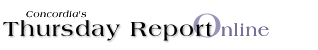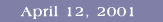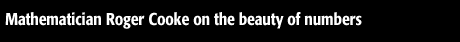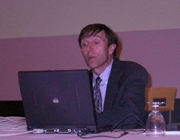Roger Cooke by Professor M.E. Szabo In the last lecture of the Science College Public lecture series for 2000/2001, Professor Roger Cooke, of the University of Vermont, lectured on “Brahmagupta, Pythagoras, and Fibonacci” to an appreciative audience. Cooke mesmerized more than 500 students, professors and members of the general public with his fascinating account of the beauty, complexity, and relevance of numbers and patterns of numbers to the real world. He spoke of the tensions between discrete and continuous mathematics, and illustrated them with historical anecdotes about how the processes of counting and measuring have spawned very different kinds of mathematics. By considering such concrete problems as determining the cost of filling up a fuel tank, Cooke led the audience step by step to the distinction between integers and real numbers. He explained how integers are the basis of arithmetic, real numbers the basis of geometry. Money belongs to arithmetic, areas and volumes to geometry. Yet the two are frequently linked by commercial transactions such as calculating the cost of filling up a fuel tank. Professor Cooke explored the difficulties involved in such tasks as measuring the speed of light and determining the circumference of a circle, and showed why some of these tasks require the idea of infinite precision. He pointed out that one of the challenges of the world of digital computers is how problems involving infinite precision can be solved with tools that are inherently finite. In his excursions into the history of mathematics, Cooke explained the connections between the work of Brahmagupta, Pythagoras, and Fibonacci. He showed how the seventh-century Hindu mathematician Brahmagupta solved problems involving the measurement of time by applying the algorithm for finding the greatest common divisor of two integers. The talk ended with a vivid and concrete illustration of how the Fibonacci series of integers can be used to explain in mathematical terms how sunflower seeds appear to grow in graceful interwoven spirals.